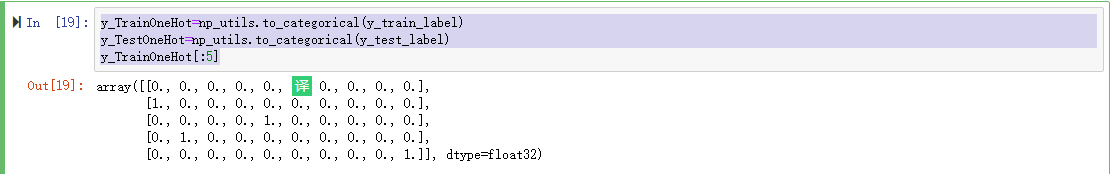# Keras手写数字识别(初识MNIST数据集)

## 1.MNIST简介

MNIST是深度学习的经典入门demo，它是由Yann LeCun搜集的，这个demo是由6万张训练图片和1万张测试图片构成的，每张图片都是28px*28px大小，而且都是黑白色构成，这些图片是采集的不同的人手写从0到9的数字。Keras将这个数据集和相关操作封装到了库中，下面我们将一步步了解MNIST的一些相关操作。

### 1.1下载MNIST数据

import numpy as np
import pandas as pd
from keras.utils import np_utils      #导入keras.utils，在后面可以将label变迁转换为One-Hot Encoding（一位有效编码）
from keras.datasets import mnist  #Keras已集成MNIST模块，可直接导入
np.random.seed(10)                     #设置seed可以产生随机数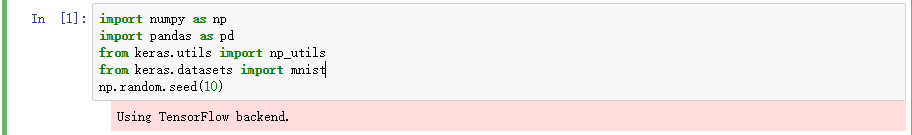(X_train_image,y_train_label),(X_test_image,y_test_label)=mnist.load_data()


X_train_image 训练样本图像
y_train_label 训练样本标签(即正确答案)
X_test_image 测试样本图像
y_test_label 测试样本答案

### 1.2查看下载的数据

print('X_train_image.shape:',X_train_image.shape)
print('y_train_label.shape:',y_train_label.shape)
print('X_test_image.shape:',X_test_image.shape)
print('y_test_label.shape:',y_test_label.shape)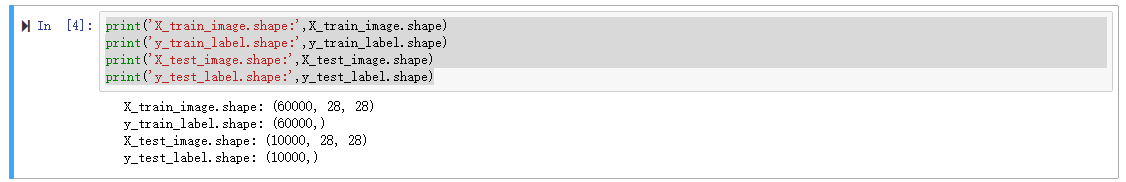import matplotlib.pyplot as plt
def plot_image(image):
fig=plt.gcf()   #gcf 返回当前Figure 对象的句柄值
fig.set_size_inches(2,2)  #用于设置图形的尺寸，单位为英寸。这里设置显示的图像大小为(2*2)
plt.imshow(image,cmap='binary')  #显示传进来的图形，cmap参数为binary，以黑白灰度显示
plt.show() 显示图像


plot_image(X_train_image)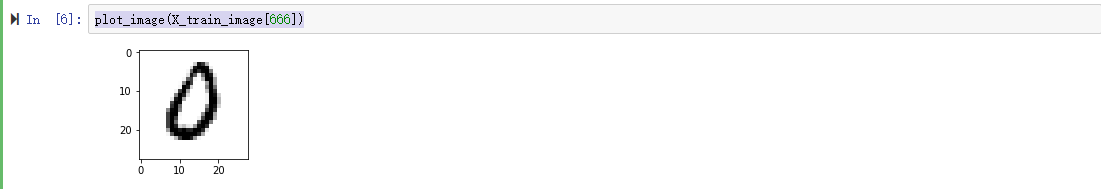y_train_label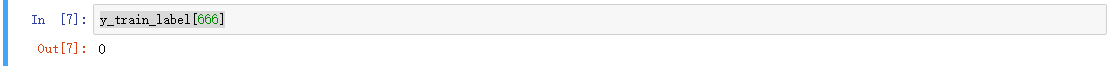### 1.3查看训练数据和测试数据的images和label

def plot_images_labels_prediction(images,labels,prediction,idx,num=10):    #idx是索引，num是默认显示10个图形
fig=plt.gcf()
fig.set_size_inches(12,14)
if num>25:                                                       #暂时设置最多显示25个图像
num=25
for i in range(0,num):
ax=plt.subplot(5,5,1+i)                                      #建立一个子图形，大小为5行5列，1+i代表在子图形中的第几个位置
ax.imshow(images[idx],cmap='binary')
title="label="+str(labels[idx])                            #定义标题样式
if len(prediction)>0:                                      #由于我们还没开始训练，所以没有预测结果，这里设置一个判断跳过，以后还会用到这个函数
title+=",prediction="+str(prediction[idx])
ax.set_title(title,fontsize=10)                      #设置标题
ax.set_xticks([])                                    #设置x轴和y轴的标签，这里设置不显示
ax.set_yticks([])
idx+=1                                               #读取下一项
plt.show()


plot_images_labels_prediction(X_train_image,y_train_label,[],0,25)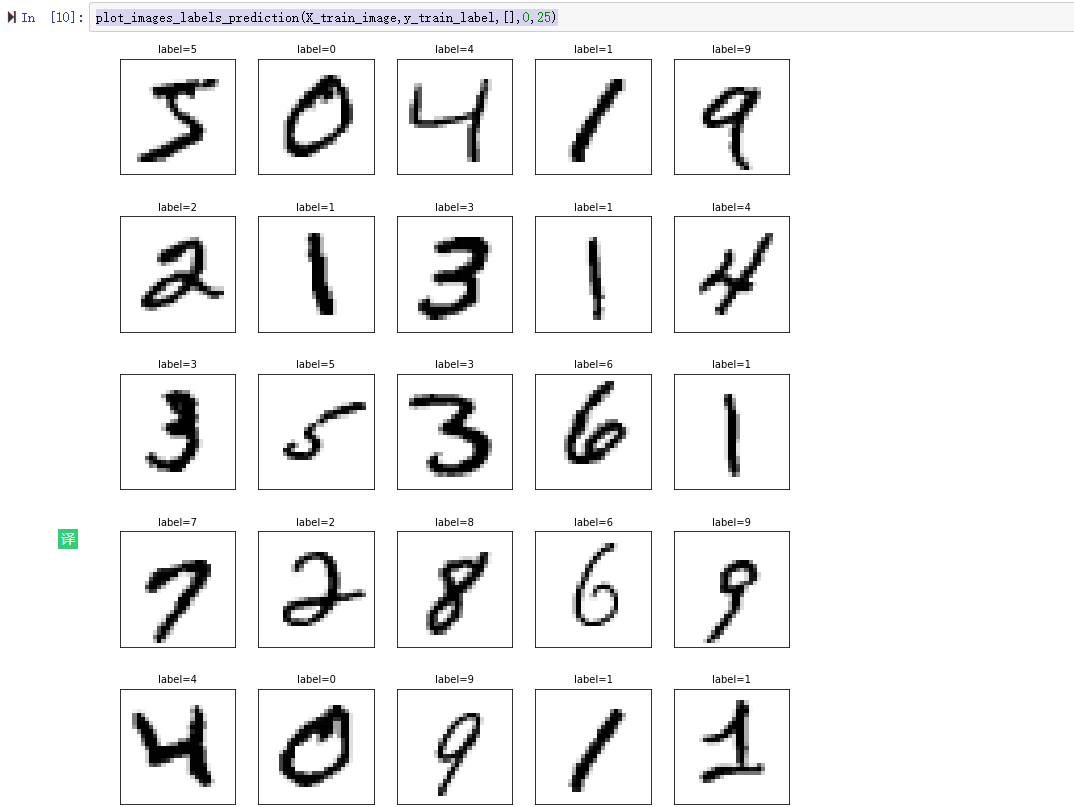plot_images_labels_prediction(X_test_image,y_test_label,[],0,29)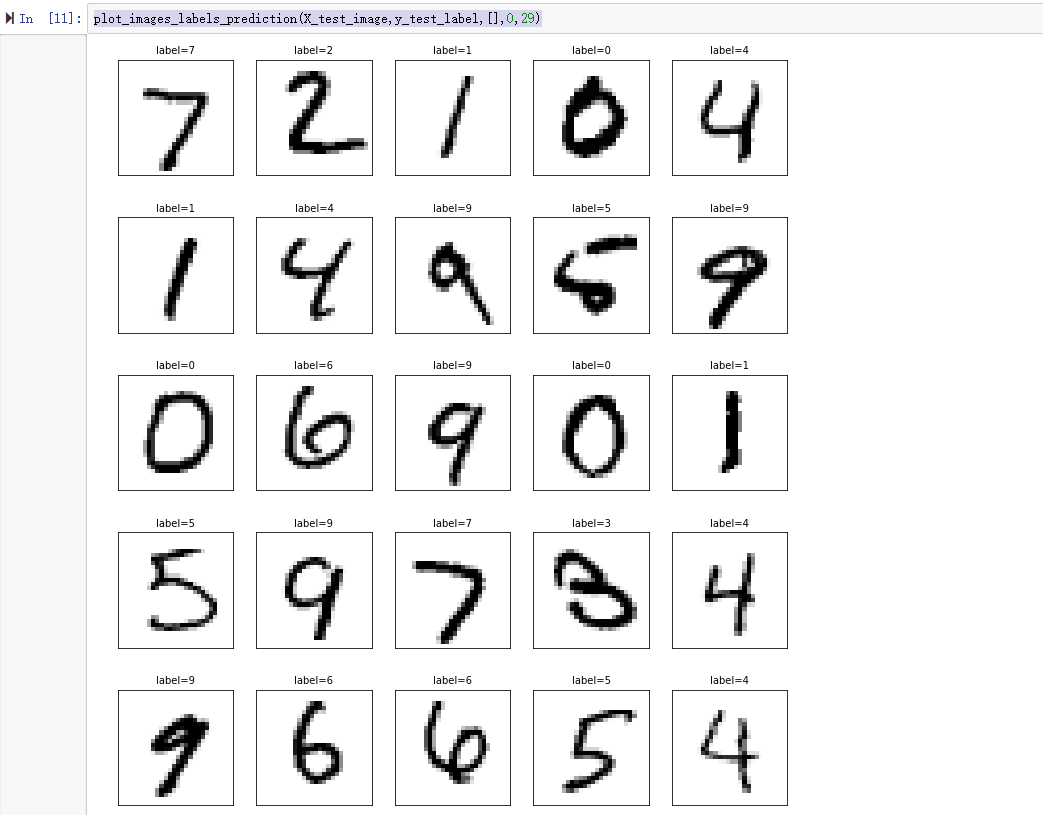### 1.4特征值预处理

X_Train=X_train_image.reshape(60000,784).astype('float32')
X_Test=X_test_image.reshape(10000,784).astype('float32')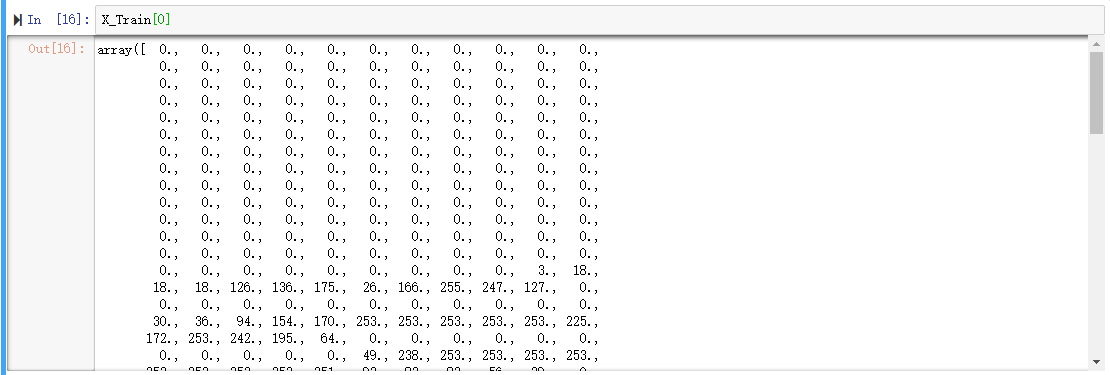X_Train_normalize=X_Train/255
X_Test_normalize=X_Test/255


X_Train_normalize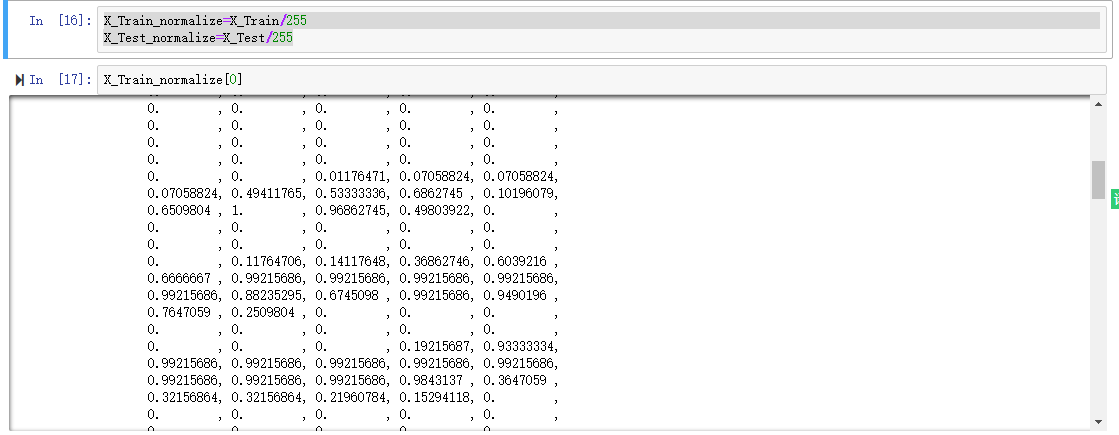### 1.5标签数据预处理

label(数字图像真实的值)标签原本是0～9的数字，必须以One-Hot Encoding（一位有效编码）转换为10个0或1的组合，例如数字5经过转换后是0000010000，正好对应输出层的10个神经元。

y_train_label[:5]y_TrainOneHot=np_utils.to_categorical(y_train_label)
y_TestOneHot=np_utils.to_categorical(y_test_label)
y_TrainOneHot[:5]